## 1、二分查找法（非递归）

1. 二分查找法只适用于从有序的数列中进行查找（比如数字和字母等），将数列排序后再进行查找

2. 二分查找法的运行时间为对数时间 O(㏒₂n) ，即查找到需要的目标位置最多只需要 ㏒₂n 步

假设从 [0,99] 的队列（100 个数，即 n=100）中寻到目标数30，则需要查找步数为㏒₂100 , 即最多需要查找 7 次（2^6 < 100 < 2^7）

public class BinarySearchNoRecur {

public static void main(String[] args) {
int[] arr = {1, 3, 8, 10, 11, 67, 100};
int index = binarySearch(arr, 11);
System.out.println("index=" + index);
}

/***
* 二分查找的非递归实现
*
* @param arr 带查找的数组
* @param target 目标数
* @return 返回对应的下标，-1代表没有找到
*/
public static int binarySearch(int arr[], int target) {
// 左边界
int left = 0;
// 右边界
int right = arr.length - 1;
while (left <= right) {
// 中间值
int mid = (left + right) / 2;
if (arr[mid] == target) {
return mid;
} else if (arr[mid] > target) {
right = mid - 1;
} else {
left = mid + 1;
}
}
return -1;
}
}

## 2、分治算法

1. 分解（Divide）：将问题划分为一些子问题，子问题的形式与原问题一样，只是规模更小
2. 解决（Conquer）：递归地求解出子问题。如果子问题规模足够小，则停止递归，直接求解
3. 合并（Combine）：将子问题的解合并为原问题的解

1. 当 n = 1 时，直接将盘子从 A → C

2. 当 n > 1 时，可以拆分为 3 步（步骤 1、3是递归调用）：

• 将 n - 1 个盘子从 A → B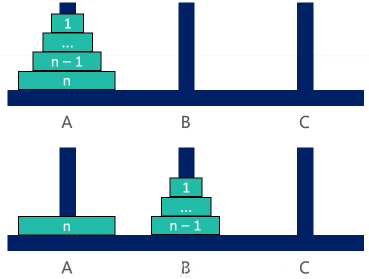• 将编号为 n 的盘子从 A → C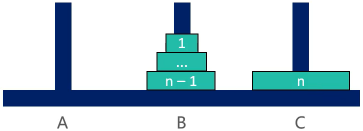• 将 n - 1 个盘子从 B→ C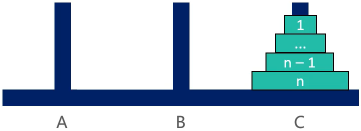public class Hanoitower {

public static void main(String[] args) {
hanoiTower(5, 'A', 'B', 'C');
}

/**
* 汉诺塔移动方法
* @param num 几个汉诺塔元素
* @param a 从哪个塔移动
* @param b 移动过程中的中间塔
* @param c 移动到的最终位置目标塔
*/
public static void hanoiTower(int num, char a, char b, char c) {
if (num == 1) {
// 如果只有一个盘子
System.out.println("第 1 个盘子从 " + a + " -> " + c);
} else {
// 如果有 n ≥ 2 ，可以看做是两个盘子：①最下面的一个盘子；②上面的所有盘子
// 1.先把最上面的所有盘 A -> B，移动过程会使用到 C
hanoiTower(num - 1, a, b, c);
// 2.把最下边的盘 A -> C
System.out.println("第 " + num + " 个盘子从 " + a + " -> " + c);
// 3.把 B 塔的所有盘从 B -> C，移动过程使用到 A 塔
hanoiTower(num - 1, b, a, c);
}
}
}

## 3、动态规划算法

1. 动态规划（Dynamic Programming）算法的核心思想是：将大问题划分为小问题进行解决，从而一步步获取最优解的处理算法
2. 动态规划算法与分治算法类似，其基本思想也是将待求解问题分解成若干个子问题，先求解子问题，然后从这些子问题的解得到原问题的解。
3. 与分治法不同的是，适合于用动态规划求解的问题，经分解得到子问题往往不是互相独立的（即下一个子阶段的求解是建立在上一个子阶段的解的基础上，进行进一步的求解）
4. 动态规划可以通过填表的方式来逐步推进，得到最优解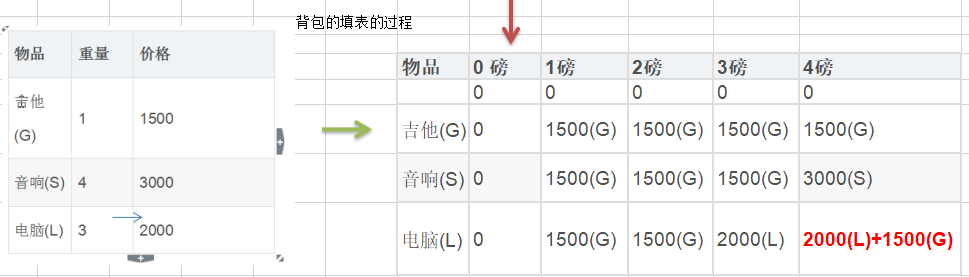• weight(row-1)：当前准备新增商品的重量
• value(row-1)：当前准备新增商品的价值
• col-weight[row-1]：加入新增产品后背包剩余的可用重量
• form[row-1][col-weight[row-1]]：在当前表格的上一行中，磅数=新增产品后背包剩余的可用重量时的装配出来的总价值

public class KnapsackProblem {

public static void main(String[] args) {
int[] weight = {1, 4, 3}; // 物品重量
int[] value = {1500, 3000, 2000}; // 物品的价值
int capacity = 4; // 背包的容量
int num = value.length; // 物品的个数

int[][] form = new int[num+1][capacity+1]; // 记录的表格
int[][] result = new int[num+1][capacity+1]; // 记录放入商品的情况

// 初始化第一行和第一列数据
for (int i = 0; i < form.length; i++) {
form[i] = 0;
}
for (int i = 0; i < form.length; i++) {
form[i] = 0;
}

// 不处理第0行和第0列
for (int row = 1; row < form.length; row++) {
for (int col = 1; col < form.length; col++) {
// 如果第一个物品重量 ＞ 当前列数（也就是磅数）
if (weight[row-1] > col) {
// 当准备加入新增的商品的容量大于当前背包的容量时，就直接使用上一个单元格的装入策略
form[row][col] = form[row-1][col];
} else {
// 进入到该处说明：准备加入的新增商品的容量小于等于当前背包的容量
if (form[row-1][col] < value[row-1] + form[row-1][col-weight[row-1]]) {
form[row][col] = value[row-1] + form[row-1][col-weight[row-1]];
result[row][col] = 1;
} else {
form[row][col] = form[row-1][col];
}
}

System.out.printf("\n处理了第 %d 行 第 %d 列 后的结果：\n", row, col);
// 输出表格
for(int i =0; i < form.length;i++) {
for(int j = 0; j < form[i].length;j++) {
System.out.printf("| %d ", form[i][j]);
}
System.out.println("|");
}
}
}

System.out.println("最优解：");
int i = result.length - 1; //行的最大下标
int j = result.length - 1;  //列的最大下标
while(i > 0 && j > 0 ) { //从 reuslt 的最后开始找
if(result[i][j] == 1) {
System.out.printf("第%d个商品放入到背包\n", i);
j -= weight[i-1];
}
i--;
}
}
}

## 4、KMP 算法

KMP 算法要解决的问题就是在字符串（也叫主串）中的模式（pattern）定位问题。说简单点就是我们平时常说的关键字搜索。模式串就是关键字（接下来称它为 P），如果它在一个主串（接下来称为 T）中出现，就返回它的具体位置，否则返回-1（常用手段）

1. 首先，使用 str1 的第一个字符和 str2 的第一个字符去比较，不符合，关键词向后移动一位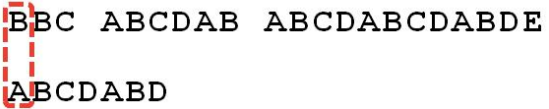2. 重复第一步，还是不符合，再后移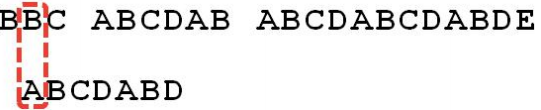3. 一直重复，直到 str1 有一个字符与 str2 的第一个字符符合为止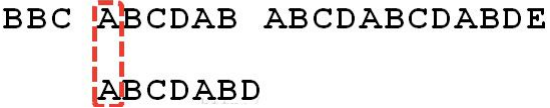4. 接着比较字符串和搜索词的下一个字符，还是符合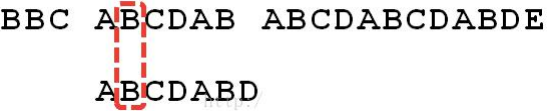5. 遇到 str1 有一个字符与 str2 对应的字符不符合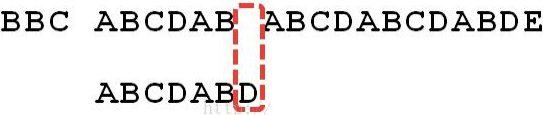6. 这时候，想到的是继续遍历 str1 的下一个字符，重复第一步（其实不好，因为当空格与 D 不匹配时，已经知道前六个字符是“ABCDAB”）。

KMP 算法的想法是，设法利用这个已知信息，不要把“搜索位置”移回已经比较过的位置，继续把它向后移，这个就提高了效率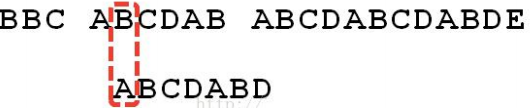7. 如何把刚刚重复的步骤省略屌，可以对 str2 计算出一张《部分匹配表》（后面介绍）8. 已知空格与 D 不匹配，前面六个字符”ABCDAB“是匹配的。查表可知，最后一个匹配字符 B 对应的”部分匹配值“为2，因此按照下面的公式算出向后移动的位数：

• 移动位数 = 已匹配的字符数 - 对应部分匹配值

因为 6-2=4，所以将搜索词向后移动 4 位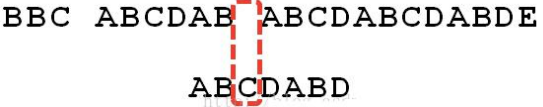9. 因为空格与 C 不匹配，搜索词还要继续往后移。这时，已匹配的字符数为 2（“AB”），对应的“部分匹配值”为0.因此，移动位数 = 2-0，将搜索词向后移动 2 位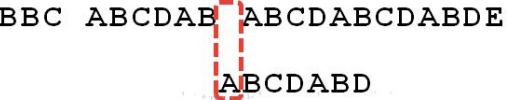10. 因为空格与 A 不匹配，继续后移一位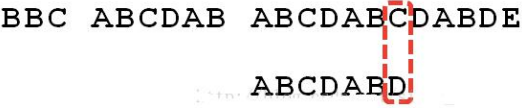11. 逐位比较，直到发现 C 与 D 不匹配。于是移动位数 = 6-2，将搜索词移动4位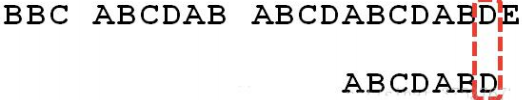12. 逐位比较，直到搜索词的最后一位，发现完全匹配，于是搜索完成，如果还要继续搜索（即找出全部匹配），移动位数 = 7-0.将搜索词向后移动 7 位“部分匹配值”就是”前缀”和”后缀”的最长的共有元素的长度。以”ABCDABD”为例，

• ”A”的前缀和后缀都为空集，共有元素的长度为 0；

• ”AB”的前缀为 [A]，后缀为 [B]，共有元素的长度为 0；

• ”ABC”的前缀为 [A,AB]，后缀为 [BC, C]，共有元素的长度 0；

• ”ABCD”的前缀为 [A,AB, ABC]，后缀为[ BCD, CD, D]，共有元素的长度为 0；

• ”ABCDA”的前缀为 [A,AB, ABC, ABCD]，后缀为 [BCDA, CDA, DA, A]，共有元素为”A”，长度为 1；

• ”ABCDAB”的前缀为 [A,AB, ABC, ABCD, ABCDA]，后缀为 [BCDAB, CDAB, DAB, AB, B]，共有元素为”AB”，长度为 2；

• ”ABCDABD”的前缀为 [A,AB, ABC, ABCD, ABCDA, ABCDAB]，后缀为 [BCDABD, CDABD, DABD, ABD, BD,D]，共有元素的长度为 0。

“部分匹配”的实质是，有时候，字符串头部和尾部会有重复。比如“ABCDAB”中有两个“AB”，那么它的“部分匹配值”就是2（“AB”的长度）。搜索词移动的时候，第一个“AB”向后移动 4 位（字符串长度 - 部分匹配值），就可以来到第二个“AB”的位置

public class KMPAlgorithm {

public static void main(String[] args) {
String str1 = "BBC ABCDAB ABCDABCDABDE";
String str2 = "ABCDABD";

int[] next = kmpNext("ABCDABD"); //[0, 1, 2, 0]
System.out.println("部分匹配表=" + Arrays.toString(next));

int index = kmpSearch(str1, str2, next);
System.out.println("index=" + index); // 15了
}

/**
* kmp 搜索算法
* @param str1 源字符串
* @param str2 子串
* @param next 部分匹配表, 是子串对应的部分匹配表
* @return 如果是-1就是没有匹配到，否则返回第一个匹配的位置
*/
public static int kmpSearch(String str1, String str2, int[] next) {
for(int i = 0, j = 0; i < str1.length(); i++) {
while( j > 0 && str1.charAt(i) != str2.charAt(j)) {
j = next[j-1];
}

if(str1.charAt(i) == str2.charAt(j)) {
j++;
}
if(j == str2.length()) { // 找到了
return i - j + 1;
}
}
return  -1;
}

/**
* 获取子串的部分匹配表
* @param dest 子串
* @return 子串的部分匹配表
*/
public static  int[] kmpNext(String dest) {
// 创建一个 next 数组保存部分匹配值
int[] next = new int[dest.length()];
next = 0; // 如果字符串是长度为1 部分匹配值就是0
for(int i = 1, j = 0; i < dest.length(); i++) {
// 当 dest.charAt(i) != dest.charAt(j) ，我们需要从 next[j-1] 获取新的 j
// 直到我们发现 有 dest.charAt(i) == dest.charAt(j) 成立才退出
// 这是 kmp 算法的核心点
while(j > 0 && dest.charAt(i) != dest.charAt(j)) {
j = next[j-1];
}
// 当 dest.charAt(i) == dest.charAt(j) 满足时，部分匹配值就是+1
if(dest.charAt(i) == dest.charAt(j)) {
j++;
}
next[i] = j;
}
return next;
}
}

public class ViolenceMatch {

public static void main(String[] args) {
//测试暴力匹配算法
String str1 = "瓦达西瓦，精神小伙！oh,my baby,fuck your mother every day";
String str2 = "！oh ";
int index = violenceMatch(str1, str2);
System.out.println("index=" + index);

}

/**
* 暴力匹配算法实现
* @param str1 要从这个字符串中去判断（母）
* @param str2 是否包含的字符串（子）
* @return 如果存在返回第一次出现的位置，如果没有返回 -1
*/
public static int violenceMatch(String str1, String str2) {
char[] s1 = str1.toCharArray();
char[] s2 = str2.toCharArray();

int s1Len = s1.length;
int s2Len = s2.length;

int i = 0; // i索引指向s1
int j = 0; // j索引指向s2
while (i < s1Len && j < s2Len) { // 保证匹配时不越界
if(s1[i] == s2[j]) { // 匹配ok
i++;
j++;
} else {
// 没有匹配成功，则回溯到上一位置
i = i - (j - 1);
j = 0;
}
}

//判断是否匹配成功
if(j == s2Len) {
return i - j;
} else {
return -1;
}
}
}

## 5、贪心算法

1. 贪心算法（贪婪算法）是指对问题进行求解时，在每一步选择中都才去最好或者最优的选择
2. 贪心算法所得到的的结果不一定是最优结果，但都是相对近似（接近）最优解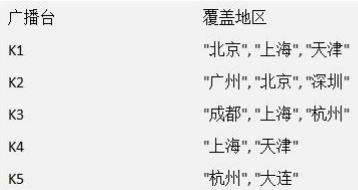1. 列举出所有城市，放入一个集合中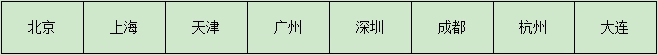2. 进行第一轮的查找，假设 k1 为覆盖地区数量值最大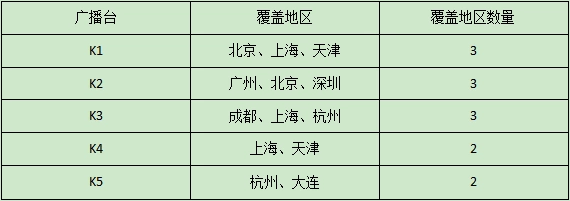3. 这时将 k1 对应的城市从集合中删除，进行下一轮比较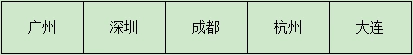4. 进行下一轮的查找，假设 k2 为覆盖地区数量值最大，重复上述步骤……

public class GreedyAlgorithm {

public static void main(String[] args) {
// 创建广播电台,放入到Map
HashMap<String, HashSet<String>> broadcasts = new HashMap<String, HashSet<String>>();

HashSet<String> hashSet1 = new HashSet<String>();

HashSet<String> hashSet2 = new HashSet<String>();

HashSet<String> hashSet3 = new HashSet<String>();

HashSet<String> hashSet4 = new HashSet<String>();

HashSet<String> hashSet5 = new HashSet<String>();

// 加入到map

// allAreas 存放所有的地区
HashSet<String> allAreas = new HashSet<String>();

// 创建ArrayList, 存放选择的电台集合
ArrayList<String> selects = new ArrayList<String>();

// 定义一个临时的集合， 在遍历的过程中，存放遍历过程中的电台覆盖的地区和当前还没有覆盖的地区的交集
HashSet<String> tempSet = new HashSet<String>();

// 定义给maxKey ， 保存在一次遍历过程中，能够覆盖最大未覆盖的地区对应的电台的key
// 如果maxKey 不为null , 则会加入到 selects
String maxKey = null;
while (allAreas.size() != 0) { // 如果 allAreas 不为 0, 则表示还没有覆盖到所有的地区
// 每进行一次 while，需要初始化
maxKey = null;

for (String key : broadcasts.keySet()) {
// 每进行一次 for
tempSet.clear();
// 当前这个 key 能够覆盖的地区
// 求出 tempSet 和 allAreas 集合的交集, 交集会赋给 tempSet
tempSet.retainAll(allAreas);
// 如果当前这个集合包含的未覆盖地区的数量，比 maxKey 指向的集合地区还多，就需要重置 maxKey
if (tempSet.size() > 0 &&
(maxKey == null || tempSet.size() > broadcasts.get(maxKey).size())) {
maxKey = key;
}
}
// maxKey != null, 就应该将 maxKey 加入 selects
if (maxKey != null) {
//将 maxKey 指向的广播电台覆盖的地区，从 allAreas 去掉
}
}

System.out.println("得到的选择结果是" + selects);// [K1,K2,K3,K5]
}
}

## 7、克鲁斯卡尔算法

1. 克鲁斯卡尔（Kruskal）算法，是用来求加权连通图的最小生成树的算法。
2. 基本思想：按照权值从小到大的顺序选择 n-1 条边，并保证这 n-1 条边不构成回路
3. 具体做法：首先构造一个只含 n 个顶点的森林，然后依权值从小到大从连通网中选择边加入到森林中，并使森林中不产生回路，直至森林变成一棵树为止

1. 对图的所有边按照权值大小进行排序

2. 将边添加到最小生成树中时，判断是否形成了回路

记录顶点在“最小生成树”中的终点，顶点的终点是“在最小生成树中与它连通的最大顶点”、然后每次需要将一条边添加到最小生成树中，判断该边的两个顶点的重点是否重合，重合的话则会构成回路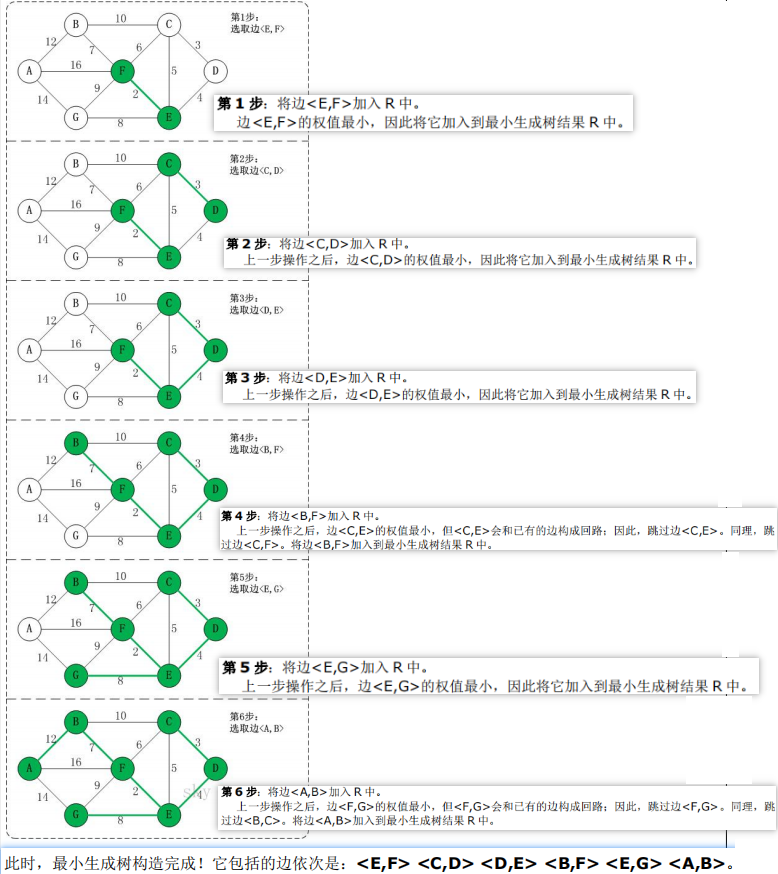public class KruskalCase {

private int edgeNum;    // 边的个数
private char[] vertexs; // 顶点数组
private int[][] matrix; // 邻接矩阵
// 使用 INF 表示两个顶点不能连通
private static final int INF = Integer.MAX_VALUE;

public static void main(String[] args) {
char[] vertexs = {'A', 'B', 'C', 'D', 'E', 'F', 'G'};
// 克鲁斯卡尔算法的邻接矩阵
int matrix[][] = {
/*A*//*B*//*C*//*D*//*E*//*F*//*G*/
/*A*/ {   0,  12, INF, INF, INF,  16,  14},
/*B*/ {  12,   0,  10, INF, INF,   7, INF},
/*C*/ { INF,  10,   0,   3,   5,   6, INF},
/*D*/ { INF, INF,   3,   0,   4, INF, INF},
/*E*/ { INF, INF,   5,   4,   0,   2,   8},
/*F*/ {  16,   7,   6, INF,   2,   0,   9},
/*G*/ {  14, INF, INF, INF,   8,   9,   0}
};
// 创建KruskalCase 对象实例
KruskalCase kruskalCase = new KruskalCase(vertexs, matrix);
// 输出构建的
kruskalCase.print();

EData[] edges = kruskalCase.getEdges();
System.out.println("排序前：" + Arrays.toString(edges));
kruskalCase.sortEdges(edges);
System.out.println("排序后：" + Arrays.toString(edges));

kruskalCase.kruskal();
}

/**
* 构造器方法
*
* @param vertexs 顶点数组
* @param matrix  邻接矩阵
*/
public KruskalCase(char[] vertexs, int[][] matrix) {
// 初始化顶点数和边的个数
int vlen = vertexs.length;

// 初始化顶点, 复制拷贝的方式
this.vertexs = new char[vlen];
for (int i = 0; i < vertexs.length; i++) {
this.vertexs[i] = vertexs[i];
}

// 初始化边, 使用的是复制拷贝的方式
this.matrix = new int[vlen][vlen];
for (int i = 0; i < vlen; i++) {
for (int j = 0; j < vlen; j++) {
this.matrix[i][j] = matrix[i][j];
}
}
// 统计边的条数
for (int i = 0; i < vlen; i++) {
for (int j = i + 1; j < vlen; j++) {
if (this.matrix[i][j] != INF) {
edgeNum++;
}
}
}
}

/**
* 打印邻接矩阵
*/
public void print() {
System.out.println("邻接矩阵为: \n");
for (int i = 0; i < vertexs.length; i++) {
for (int j = 0; j < vertexs.length; j++) {
System.out.printf("%12d", matrix[i][j]);
}
System.out.println();//换行
}
}

/**
* 对边进行冒泡排序
*
* @param edges 边的集合
*/
public void sortEdges(EData[] edges) {
for (int i = 0; i < edges.length - 1; i++) {
for (int j = -0; j < edges.length - 1 - i; j++) {
if (edges[j].weight > edges[j+1].weight) {
EData temp = edges[j];
edges[j] = edges[j+1];
edges[j+1] = temp;
}
}
}
}

/**
*
* @param ch 顶点的值，例如'A'
* @return 返回 ch 顶点对应的下标，如果找不到返回-1
*/
private int getPosition(char ch) {
for (int i = 0; i < vertexs.length; i++) {
if (vertexs[i] == ch) {
return i;
}
}
return -1;
}

/**
* 获取图中边，放到 EData[] 数组中，后面需要遍历该数组
* 通过 matrix 邻接矩阵来获取
*
* @return 例如：[['A', 'B', 12], ['D', 'F', 7], ...]
*/
private EData[] getEdges() {
int index = 0;
EData[] edges = new EData[edgeNum];
for (int i = 0; i < vertexs.length; i++) {
for (int j = i+1; j < vertexs.length; j++) {
if (matrix[i][j] != INF) {
edges[index++] = new EData(vertexs[i], vertexs[j], matrix[i][j]);
}
}
}
return edges;
}

/**
* 功能: 获取下标为 i 的顶点的终点(), 用于后面判断两个顶点的终点是否相同
*
* @param ends 数组就是记录了各个顶点对应的终点是哪个，ends 数组是在遍历过程中，逐步形成
* @param i    表示传入的顶点对应的下标
* @return 返回的就是 下标为i的这个顶点对应的终点的下标, 一会回头还有来理解
*/
private int getEnd(int[] ends, int i) { // i = 4 [0,0,0,0,5,0,0,0,0,0,0,0]
while (ends[i] != 0) {
i = ends[i];
}
return i;
}

/**+
* 克鲁斯卡尔算法
*/
public void kruskal() {
int index = 0; // 表示最后结果数组的索引
int[] ends = new int[edgeNum]; // 用于保存"已有最小生成树" 中的每个顶点在最小生成树中的终点
// 创建结果数组, 保存最后的最小生成树
EData[] rets = new EData[edgeNum];

// 获取图中 所有的边的集合 ， 一共有12边
EData[] edges = getEdges();
System.out.println("图的边的集合=" + Arrays.toString(edges) + " 共" + edges.length); // 12

// 按照边的权值大小进行排序（从小到大）
sortEdges(edges);

// 遍历 edges 数组，将边添加到最小生成树中时，判断是准备加入的边否形成了回路，如果没有，就加入 rets, 否则不能加入
for (int i = 0; i < edgeNum; i++) {
// 获取到第 i 条边的第1个顶点(起点)
int p1 = getPosition(edges[i].start); // p1 = 4
// 获取到第 i 条边的第2个顶点
int p2 = getPosition(edges[i].end); // p2 = 5

// 获取 p1 这个顶点在已有最小生成树中的终点
int m = getEnd(ends, p1); // m = 4
// 获取 p2 这个顶点在已有最小生成树中的终点
int n = getEnd(ends, p2); // n = 5
// 是否构成回路
if (m != n) { // 没有构成回路
ends[m] = n; // 设置m 在"已有最小生成树"中的终点 <E,F> [0,0,0,0,5,0,0,0,0,0,0,0]
rets[index++] = edges[i]; // 有一条边加入到 rets 数组
}
}
// 统计并打印 "最小生成树", 输出  rets
System.out.println("最小生成树为");
for (int i = 0; i < index; i++) {
System.out.println(rets[i]);
}
}
}

/**
* 创建一个类EData ，它的对象实例就表示一条边
*/
class EData {
char start; // 边的一个点
char end;   // 边的另外一个点
int weight; // 边的权值

public EData(char start, char end, int weight) {
this.start = start;
this.end = end;
this.weight = weight;
}

@Override
public String toString() {
return "EData [<" + start + ", " + end + ">= " + weight + "]";
}
}

## 8、迪杰斯特拉算法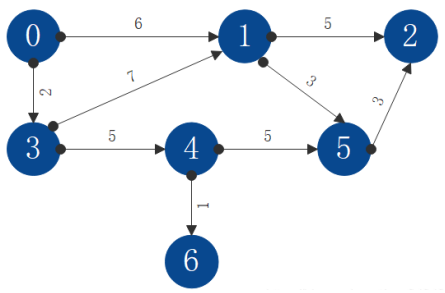S 为已求出最短路径的节点，T 为未求出最短路径的节点。源节点只允许将 S 中的节点作为中间节点来计算到达其它节点的最短路径，不允许将 T 中的节点作为中间节点来计算到达其它节点的最短路径。随着 S 中节点的增加，源节点可达的节点才会增加。初始状态下，源节点只可达节点 1 和节点 3

1. 将源节点（即节点0）加入S中，对 shortestvisited 数组进行更新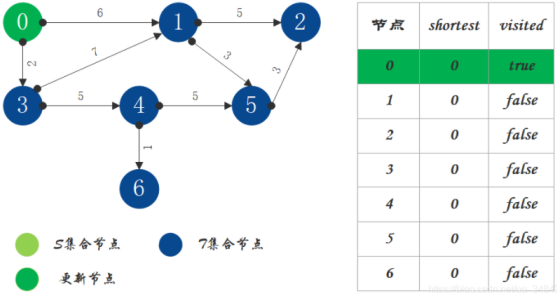2. S 中现有节点 0，源节点可达 T 中的节点 1 和节点 3，节点 0 -> 节点 1 距离为 6，节点 0 -> 节点 3 距离为 2，按距离从小到大排序，因此选择将节点 3 加入 S 中。更新源点将节点 3 作为中间节点到达其它节点的距离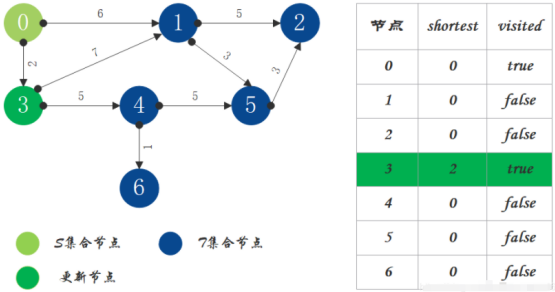3. S 中现有节点 0 和节点 3，源节点可达 T 中的节点 1 和 4，节点 0 -> 节点 1 距离为 6，节点 0 -> 节点 4 距离为 7，按距离从小到大排序，因此选择将节点 1 加入 S 中。更新源点将节点 1 作为中间节点到达其它节点的距离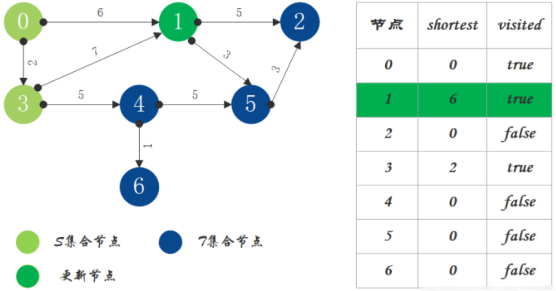4. S 中现有节点 0、1、3，源节点可达 T 中的节点 2、4、5，0 -> 2 距离为 11，0 -> 4 距离为 7，0 -> 5 距离为 9，按距离从小到大排序，因此选择将节点 4 加入 S 中。更新源点将节点 4 作为中间节点到达其它节点的距离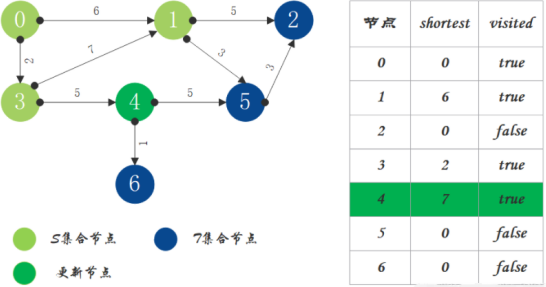5. S 中现有节点 0、1、3、4，源节点可达 T 中的节点 2、5、6，0 -> 2 距离为 11，0 -> 5 距离为 9，0 -> 6 距离为 8，按距离从小到大排序，因此选择将节点 6 加入 S 中。更新源点将节点 6 作为中间节点到达其它节点的距离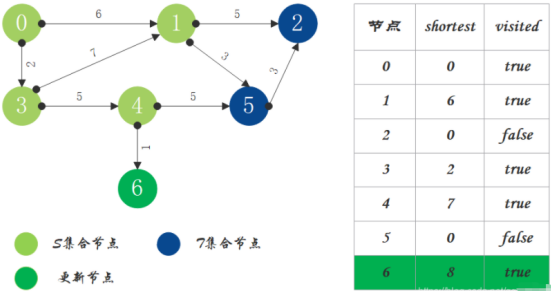6. S 中现有节点 0、1、3、4、6，源节点可达T中的节点 2、5，0 -> 2 距离为 11，0 -> 5 距离为 9，按距离从小到大排序，因此选择将节点 5 加入 S 中。更新源点将节点 5 作为中间节点到达其它节点的距离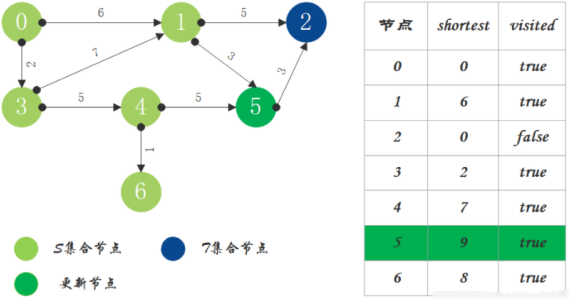7. T 中只剩下节点 2，0 -> 2 距离为 11，将节点 2 加入 S 中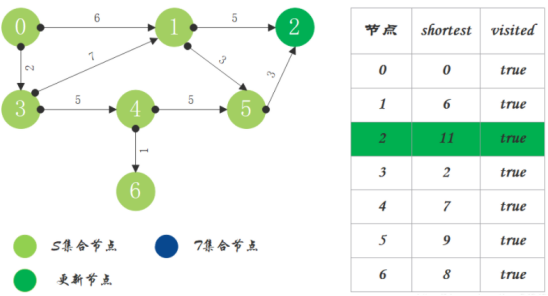8. 算法结束，源点到其它节点的最短路径都已依次求出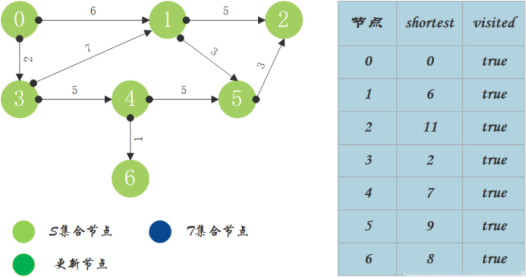public class DijstraAlgorithm {

public static int MaxValue = 100000;

/**
* 迪杰斯特拉算法
*
* @param matrix 传入图的邻接矩阵
* @param source 源节点
*/
public static void dijstra(int[][] matrix, int source) {
// 最短路径长度
int[] shorttest = new int[matrix.length];
// 判断该点最短路径是否求出
boolean[] visited = new boolean[matrix.length];
// 存储输出路径
String[] path = new String[matrix.length];

//初始化输出路径
for (int i = 0; i < matrix.length; i++) {
path[i] = new String(source + "-->" + i);
}

shorttest[source] = 0; // 初始化时将源节点 0 加入其中，源节点到源节点的距离为 0
visited[source] = true;   // 表明源节点到该节点的最短路径以求出（就是 0）

// 因为第一个节点是源节点所以下标从 1 开始，求出 0->1，0->2...的路径
for (int i = 1; i < matrix.length; i++) {
int min = Integer.MAX_VALUE;
int index = -1; // 当前节点的索引位置

// 循环找出最短路径
for (int j = 0; j < matrix.length; j++) {
// 已经求出的最短路径节点不需要再加入计算判断加入节点是否存在更短路径
if (!visited[j] && matrix[source][j] < min) {
min = matrix[source][j];
index = j;
}
}

// 更新最短路径
shorttest[index] = min;
// 更改最短状态，如果是 true 表示已经是最短了，如果是 false 表示没有找到
visited[index] = true;

// 更新 index 跳其他节点的路径
for (int m = 0; m < matrix.length; m++) {
/*
!visited[m]：当前节点最短路径暂未求出
matrix[source][index]：源点距离当前节点的最小距离
matrix[index][m]：当前节点到 m 节点的距离
matrix[source][m]：源点到 m 节点的距离
*/
if (!visited[m] && matrix[source][index] + matrix[index][m] < matrix[source][m]) {
matrix[source][m] = matrix[source][index] + matrix[index][m];
path[m] = path[index] + "-->" + m;
}
}

}

//打印最短路径
for (int i = 0; i < matrix.length; i++) {
if (i != source) {
if (shorttest[i] == MaxValue) {
System.out.println(source + "到" + i + "不可达");
} else {
System.out.println(source + "到" + i + "的最短路径为:" + path[i] + "，最短距离是:" + shorttest[i]);
}
}
}
}
}

public class MyTest {

public static void main(String[] args) {

int vertex = 7; // 顶点数
int edge = 10; // 边数
int[][] matrix = {
//      0        1         2          3       4        5         6
/*0*/  {100000,       6,  100000,       2,  100000,  100000,  100000},
/*1*/  {100000,  100000,       5,  100000,  100000,       3,  100000},
/*2*/  {100000,  100000,  100000,  100000,  100000,  100000,  100000},
/*3*/  {100000,       7,  100000,  100000,       5,  100000,  100000},
/*4*/  {100000,  100000,  100000,  100000,  100000,       5,       1},
/*5*/  {100000,  100000,  100000,  100000,       2,  100000,  100000},
/*6*/  {100000,  100000,  100000,  100000,  100000,  100000,  100000}
};

System.out.println("初始邻接矩阵： ");
for (int i = 0; i < matrix.length; i++) {
for (int j = 0; j < matrix.length; j++) {
System.out.printf("%12d", matrix[i][j]);
}
System.out.println();
}

int source = 0;
DijstraAlgorithm.dijstra(matrix, source);

System.out.println("迪杰斯特拉算法后邻接矩阵：");
for (int i = 0; i < matrix.length; i++) {
for (int j = 0; j < matrix.length; j++) {
System.out.printf("%12d", matrix[i][j]);
}
System.out.println();
}
}
}

## 9、佛洛依德算法

• 迪杰斯特拉算法通过选定的被访问顶点，求出从访问顶点到其他顶点的最短路径
• 佛洛依德算法中过每一个顶点都是触发访问点，所以需要将每一个顶点看做被访问顶点，求出每一个顶点到其他顶点的最短路径

1. 初始化，根据示例图构建距离表（N代表无限远不可到达）和前驱表
2. 从 A 开始，查找以 A 为中间顶点的路线，C-A-G, C-A-B, G-A-B
3. 对比距离表，由于 C-A-G，即 C 到达 G 的距离为 9，比图中 C-G 的距离 N 小，则修改距离表的距离并且修改前驱表的中间结点为 A
4. 依次类推，遍历每一个字符当作中间结点，比较两点的直接距离和存在中间结点的距离，选择最小的那个当作距离结果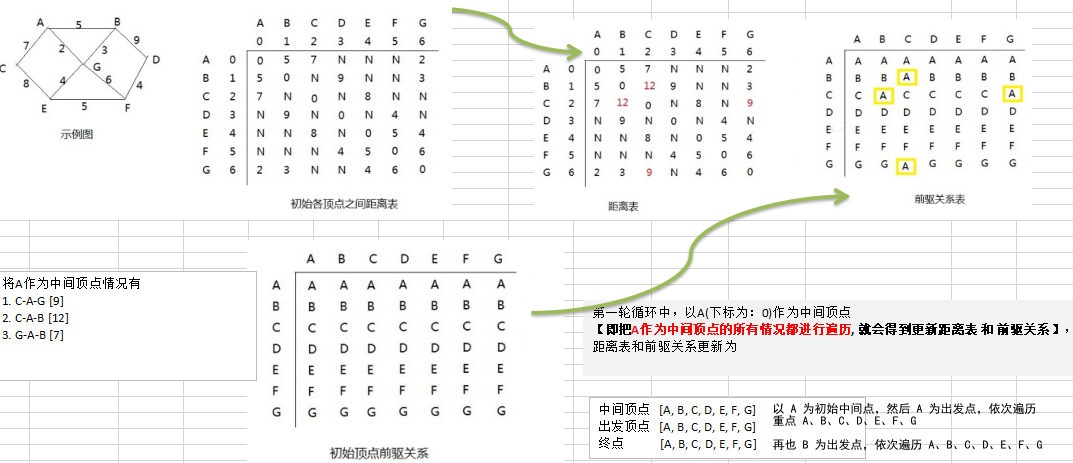public class FloydAlgorithm {

public static void main(String[] args) {
// 测试看看图是否创建成功
char[] vertex = {'A', 'B', 'C', 'D', 'E', 'F', 'G'};
//创建邻接矩阵
int[][] matrix = new int[vertex.length][vertex.length];
final int N = 65535;
matrix = new int[]{0, 5, 7, N, N, N, 2};
matrix = new int[]{5, 0, N, 9, N, N, 3};
matrix = new int[]{7, N, 0, N, 8, N, N};
matrix = new int[]{N, 9, N, 0, N, 4, N};
matrix = new int[]{N, N, 8, N, 0, 5, 4};
matrix = new int[]{N, N, N, 4, 5, 0, 6};
matrix = new int[]{2, 3, N, N, 4, 6, 0};

//创建 Graph 对象
Graph graph = new Graph(vertex.length, matrix, vertex);
//调用弗洛伊德算法
graph.floyd();
graph.show();
}

}

// 创建图
class Graph {
private char[] vertex;  // 存放顶点的数组
private int[][] dis;    // 保存，从各个顶点出发到其它顶点的距离，最后的结果，也是保留在该数组
private int[][] pre;    // 保存到达目标顶点的前驱顶点

/**
* 构造器
*
* @param length 大小
* @param matrix 邻接矩阵
* @param vertex 顶点数组
*/
public Graph(int length, int[][] matrix, char[] vertex) {
this.vertex = vertex;
this.dis = matrix;
this.pre = new int[length][length];
// 对pre数组初始化, 注意存放的是前驱顶点的下标
for (int i = 0; i < length; i++) {
Arrays.fill(pre[i], i);
}
}

/**
* 显示pre数组和dis数组
*/
public void show() {
// 为了显示便于阅读，优化一下输出
char[] vertex = {'A', 'B', 'C', 'D', 'E', 'F', 'G'};
for (int k = 0; k < dis.length; k++) {
// 先将pre数组输出的一行
for (int i = 0; i < dis.length; i++) {
System.out.print(vertex[pre[k][i]] + " ");
}
System.out.println();
// 输出dis数组的一行数据
for (int i = 0; i < dis.length; i++) {
System.out.print("(" + vertex[k] + "到" + vertex[i] + "的最短路径是" + dis[k][i] + ") ");
}
System.out.println("\n");
}
}

/**
* 佛洛依德算法
*/
public void floyd() {
int len = 0; // 变量保存距离
// 对中间顶点遍历， k 就是中间顶点的下标 [A, B, C, D, E, F, G]
for (int k = 0; k < dis.length; k++) { //
// 从 i 顶点开始出发 [A, B, C, D, E, F, G]
for (int i = 0; i < dis.length; i++) {
// 到达 j 顶点 // [A, B, C, D, E, F, G]
for (int j = 0; j < dis.length; j++) {
len = dis[i][k] + dis[k][j];// 求出从 i 顶点出发，经过 k 中间顶点，到达 j 顶点距离
if (len < dis[i][j]) {  // 如果len小于 dis[i][j]
dis[i][j] = len;    // 更新距离
pre[i][j] = pre[k][j];// 更新前驱顶点
}
}
}
}
}
}

## 10、马踏棋盘算法

1. 创建棋盘 chessBoard , 是一个二维数组
2. 将当前位置设置为已经访问，然后根据当前位置，计算马儿还能走哪些位置，并放入到一个集合 ArrayList 中， 最多有 8 个位置， 每走一步，就使用 step+1
3. 遍历 ArrayList 中存放的所有位置，看看哪个可以走通 , 如果走通，就继续，走不通，就回溯
4. 判断马儿是否完成了任务，使用 step 和应该走的步数比较，如果没有达到数量，则表示没有完成任务，将整个棋盘置 0

public class HorseChessboard {

private static int X; // 棋盘的列数
private static int Y; // 棋盘的行数
//创建一个数组，标记棋盘的各个位置是否被访问过
private static boolean visited[];
//使用一个属性，标记是否棋盘的所有位置都被访问
private static boolean finished; // 如果为 true，表示成功

public static void main(String[] args) {
System.out.println("骑士周游算法，开始运行~~");
X = 8;
Y = 8;
int row = 1;    // 马儿初始位置的行，从1开始编号
int column = 1; // 马儿初始位置的列，从1开始编号
// 创建棋盘
int[][] chessboard = new int[X][Y];
visited = new boolean[X * Y]; // 初始值都是 false
// 测试一下耗时
long start = System.currentTimeMillis();
traversalChessboard(chessboard, row - 1, column - 1, 1);
long end = System.currentTimeMillis();
System.out.println("共耗时: " + (end - start) + " 毫秒");

//输出棋盘的最后情况
for (int[] rows : chessboard) {
for (int step : rows) {
System.out.print(step + "\t");
}
System.out.println();
}
}

/**
* 根据当前位置（Point对象），计算马儿还能走哪些位置（Point），并放入到一个集合中（ArrayList）, 最多有8个位置
*
* @param curPoint 当前位置
* @return
*/
public static ArrayList<Point> next(Point curPoint) {
// 创建一个ArrayList
ArrayList<Point> ps = new ArrayList<Point>();
// 创建一个Point
Point p1 = new Point();
// 表示马儿可以走 5 这个位置
if ((p1.x = curPoint.x - 2) >= 0 && (p1.y = curPoint.y - 1) >= 0) {
}
// 判断马儿可以走 6 这个位置
if ((p1.x = curPoint.x - 1) >= 0 && (p1.y = curPoint.y - 2) >= 0) {
}
// 判断马儿可以走 7 这个位置
if ((p1.x = curPoint.x + 1) < X && (p1.y = curPoint.y - 2) >= 0) {
}
// 判断马儿可以走 0 这个位置
if ((p1.x = curPoint.x + 2) < X && (p1.y = curPoint.y - 1) >= 0) {
}
// 判断马儿可以走 1 这个位置
if ((p1.x = curPoint.x + 2) < X && (p1.y = curPoint.y + 1) < Y) {
}
// 判断马儿可以走 2 这个位置
if ((p1.x = curPoint.x + 1) < X && (p1.y = curPoint.y + 2) < Y) {
}
// 判断马儿可以走 3 这个位置
if ((p1.x = curPoint.x - 1) >= 0 && (p1.y = curPoint.y + 2) < Y) {
}
// 判断马儿可以走 4 这个位置
if ((p1.x = curPoint.x - 2) >= 0 && (p1.y = curPoint.y + 1) < Y) {
}
return ps;
}

/**
* 完成骑士周游问题的算法
*
* @param chessboard 棋盘
* @param row        马儿当前的位置的行从0开始
* @param column     马儿当前的位置的列从0开始
* @param step       是第几步，初始位置就是第1步
*/
public static void traversalChessboard(int[][] chessboard, int row, int column, int step) {
chessboard[row][column] = step;
visited[row * X + column] = true; //标记该位置已经访问
// 获取当前位置可以走的下一个位置的集合
ArrayList<Point> ps = next(new Point(column, row));
// 遍历 ps
while (!ps.isEmpty()) {
Point p = ps.remove(0); // 取出下一个可以走的位置
// 判断该点是否已经访问过
if (!visited[p.y * X + p.x]) { // 说明还没有访问过
traversalChessboard(chessboard, p.y, p.x, step + 1);
}
}
// 判断马儿是否完成了任务，使用 step 和应该走的步数比较，如果没有达到数量，则表示没有完成任务，将整个棋盘置0
// 说明: step < X * Y  成立的情况有两种
// 1. 棋盘到目前为止,仍然没有走完
// 2. 棋盘处于一个回溯过程
if (step < X * Y && !finished) {
chessboard[row][column] = 0;
visited[row * X + column] = false;
} else {
finished = true;
}
}
}

1. 根据马儿当前位置，获取下一步可以走的位置的合集 ps
2. 对 ps 中所有的 Point 位置节点进行非递减排序
• 递减，例：5, 4, 3, 2, 1
• 非递减，例：5, 4, 3, 3, 3, 2, 2, 1
3. 排序依据：当前该 point 节点下一步可以走的位置合集的长度
public class HorseChessboard {

private static int X; // 棋盘的列数
private static int Y; // 棋盘的行数
//创建一个数组，标记棋盘的各个位置是否被访问过
private static boolean visited[];
//使用一个属性，标记是否棋盘的所有位置都被访问
private static boolean finished; // 如果为 true，表示成功

public static void main(String[] args) {
System.out.println("骑士周游算法，开始运行~~");
X = 8;
Y = 8;
int row = 1;    // 马儿初始位置的行，从1开始编号
int column = 1; // 马儿初始位置的列，从1开始编号
// 创建棋盘
int[][] chessboard = new int[X][Y];
visited = new boolean[X * Y]; // 初始值都是 false
// 测试一下耗时
long start = System.currentTimeMillis();
traversalChessboard(chessboard, row - 1, column - 1, 1);
long end = System.currentTimeMillis();
System.out.println("共耗时: " + (end - start) + " 毫秒");

//输出棋盘的最后情况
for (int[] rows : chessboard) {
for (int step : rows) {
System.out.print(step + "\t");
}
System.out.println();
}
}

/**
* 根据当前位置（Point对象），计算马儿还能走哪些位置（Point），并放入到一个集合中（ArrayList）, 最多有8个位置
*
* @param curPoint 当前位置
* @return
*/
public static ArrayList<Point> next(Point curPoint) {
// 创建一个ArrayList
ArrayList<Point> ps = new ArrayList<Point>();
// 创建一个Point
Point p1 = new Point();
// 表示马儿可以走 5 这个位置
if ((p1.x = curPoint.x - 2) >= 0 && (p1.y = curPoint.y - 1) >= 0) {
}
// 判断马儿可以走 6 这个位置
if ((p1.x = curPoint.x - 1) >= 0 && (p1.y = curPoint.y - 2) >= 0) {
}
// 判断马儿可以走 7 这个位置
if ((p1.x = curPoint.x + 1) < X && (p1.y = curPoint.y - 2) >= 0) {
}
// 判断马儿可以走 0 这个位置
if ((p1.x = curPoint.x + 2) < X && (p1.y = curPoint.y - 1) >= 0) {
}
// 判断马儿可以走 1 这个位置
if ((p1.x = curPoint.x + 2) < X && (p1.y = curPoint.y + 1) < Y) {
}
// 判断马儿可以走 2 这个位置
if ((p1.x = curPoint.x + 1) < X && (p1.y = curPoint.y + 2) < Y) {
}
// 判断马儿可以走 3 这个位置
if ((p1.x = curPoint.x - 1) >= 0 && (p1.y = curPoint.y + 2) < Y) {
}
// 判断马儿可以走 4 这个位置
if ((p1.x = curPoint.x - 2) >= 0 && (p1.y = curPoint.y + 1) < Y) {
}
return ps;
}

/**
* 完成骑士周游问题的算法
*
* @param chessboard 棋盘
* @param row        马儿当前的位置的行从0开始
* @param column     马儿当前的位置的列从0开始
* @param step       是第几步，初始位置就是第1步
*/
public static void traversalChessboard(int[][] chessboard, int row, int column, int step) {
chessboard[row][column] = step;
visited[row * X + column] = true; //标记该位置已经访问
// 获取当前位置可以走的下一个位置的集合
ArrayList<Point> ps = next(new Point(column, row));
// 对 ps 进行排序,排序的规则就是对 ps 的所有的 Point 对象的下一步的位置的数目，进行非递减排序
sort(ps);
// 遍历 ps
while (!ps.isEmpty()) {
Point p = ps.remove(0); // 取出下一个可以走的位置
// 判断该点是否已经访问过
if (!visited[p.y * X + p.x]) { // 说明还没有访问过
traversalChessboard(chessboard, p.y, p.x, step + 1);
}
}
// 判断马儿是否完成了任务，使用 step 和应该走的步数比较，如果没有达到数量，则表示没有完成任务，将整个棋盘置0
// 说明: step < X * Y  成立的情况有两种
// 1. 棋盘到目前为止,仍然没有走完
// 2. 棋盘处于一个回溯过程
if (step < X * Y && !finished) {
chessboard[row][column] = 0;
visited[row * X + column] = false;
} else {
finished = true;
}
}

/**
* 根据当前这个一步的所有的下一步的选择位置，进行非递减排序, 减少回溯的次数
*
* @param ps
*/
public static void sort(ArrayList<Point> ps) {
ps.sort(new Comparator<Point>() {
@Override
public int compare(Point o1, Point o2) {
//获取到 o1 的下一步的所有位置个数
int count1 = next(o1).size();
//获取到 o2 的下一步的所有位置个数
int count2 = next(o2).size();
if (count1 < count2) {
return -1;
} else if (count1 == count2) {
return 0;
} else {
return 1;
}
}
});
}
}

END